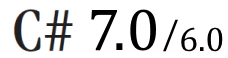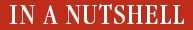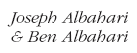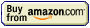# Code Listings

## Security

Permission subsets:

```PrincipalPermission jay = new PrincipalPermission ("Jay", null);
PrincipalPermission sue = new PrincipalPermission ("Sue", null);

PrincipalPermission jayOrSue = (PrincipalPermission) jay.Union (sue);
Console.WriteLine (jay.IsSubsetOf (jayOrSue));  // True```

PermissionSet:

```PermissionSet ps = new PermissionSet (PermissionState.None);

ps.AddPermission (new UIPermission (PermissionState.Unrestricted));
ps.AddPermission (new SecurityPermission (
SecurityPermissionFlag.UnmanagedCode));
ps.AddPermission (new FileIOPermission (
FileIOPermissionAccess.Read, @"c:\docs"));
ps.Demand();```

Transparency model - UnsaveXXX pattern

```[SecurityCritical]
public void UnsafeWatchTV()
{
using (Key key = GetTVRoomKey())
PrisonGuard.OpenDoor(key);
}

// The second is security-safe-critical, and calls the first after
// satisfying a full stack-walking demand:
[SecuritySafeCritical]
public void WatchTV()
{
new TVPermission().Demand();
UnsafeWatchTV();
}
```

Sandboxing another assembly:

```using System;
using System.IO;
using System.Net;
using System.Reflection;
using System.Security;
using System.Security.Policy;
using System.Security.Permissions;

class Program
{
static void Main()
{
string pluginFolder = Path.Combine (
AppDomain.CurrentDomain.BaseDirectory, "plugins");

string plugInPath = Path.Combine (pluginFolder, "plugin.exe");

PermissionSet ps = new PermissionSet (PermissionState.None);

ps.AddPermission
(new SecurityPermission (SecurityPermissionFlag.Execution));

ps.AddPermission
(new FileIOPermission (FileIOPermissionAccess.PathDiscovery |
FileIOPermissionAccess.Read, plugInPath));

ps.AddPermission (new UIPermission (PermissionState.Unrestricted));

AppDomainSetup setup = AppDomain.CurrentDomain.SetupInformation;
AppDomain sandbox = AppDomain.CreateDomain ("sbox", null, setup, ps);
sandbox.ExecuteAssembly (plugInPath);
AppDomain.Unload (sandbox);
}
}
```

Asserting permissions:

```public class Utils
{
[SecuritySafeCritical]
public static void WriteLog (string msg)
{
FileIOPermission f = new FileIOPermission (PermissionState.None);
f.AllLocalFiles = FileIOPermissionAccess.AllAccess;
f.Assert();

// Write to log
...
}
}```
```static void Main()
{
string pluginFolder = Path.Combine (
AppDomain.CurrentDomain.BaseDirectory, "plugins");

string plugInPath = Path.Combine (pluginFolder, "plugin.exe");

PermissionSet ps = new PermissionSet (PermissionState.None);

// Add desired permissions to ps as we did before
// ...

Assembly utilAssembly = typeof (Utils).Assembly;
StrongName utils = utilAssembly.Evidence.GetHostEvidence<StrongName>();

AppDomainSetup setup = AppDomain.CurrentDomain.SetupInformation;
AppDomain sandbox = AppDomain.CreateDomain ("sbox", null, setup, ps,
utils);
sandbox.ExecuteAssembly (plugInPath);
AppDomain.Unload (sandbox);
}
```

Administrative elevation and virtualization:

```<?xml version="1.0" encoding="utf-8"?>
<assembly manifestVersion="1.0" xmlns="urn:schemas-microsoft-com:asm.v1">
<trustInfo xmlns="urn:schemas-microsoft-com:asm.v2">
<security>
<requestedPrivileges>
<requestedExecutionLevel level="requireAdministrator" />
</requestedPrivileges>
</security>
</trustInfo>
</assembly>```

Cryptography: Windows data protection:

```byte[] original = {1, 2, 3, 4, 5};
DataProtectionScope scope = DataProtectionScope.CurrentUser;

byte[] encrypted = ProtectedData.Protect (original, null, scope);
byte[] decrypted = ProtectedData.Unprotect (encrypted, null, scope);
// decrypted is now {1, 2, 3, 4, 5}```

Hashing:

```byte[] hash;
using (Stream fs = File.OpenRead ("checkme.doc"))
hash = MD5.Create().ComputeHash (fs);           // hash is 16 bytes long```

or:

```byte[] data = System.Text.Encoding.UTF8.GetBytes ("stRhong%pword");
byte[] hash = SHA256.Create().ComputeHash (data);```

Symmetric encryption to a file:

```byte[] key = {145,12,32,245,98,132,98,214,6,77,131,44,221,3,9,50};
byte[] iv  = {15,122,132,5,93,198,44,31,9,39,241,49,250,188,80,7};

byte[] data = { 1, 2, 3, 4, 5 };   // This is what we're encrypting.

using (SymmetricAlgorithm algorithm = Aes.Create())
using (ICryptoTransform encryptor = algorithm.CreateEncryptor (key, iv))
using (Stream f = File.Create ("encrypted.bin"))
using (Stream c = new CryptoStream (f, encryptor, CryptoStreamMode.Write))
c.Write (data, 0, data.Length);```

Symmetric decryption from a file:

```byte[] key = {145,12,32,245,98,132,98,214,6,77,131,44,221,3,9,50};
byte[] iv  = {15,122,132,5,93,198,44,31,9,39,241,49,250,188,80,7};

byte[] decrypted = new byte;

using (SymmetricAlgorithm algorithm = Aes.Create())
using (ICryptoTransform decryptor = algorithm.CreateDecryptor (key, iv))
using (Stream f = File.OpenRead ("encrypted.bin"))
using (Stream c = new CryptoStream (f, decryptor, CryptoStreamMode.Read))
for (int b; (b = c.ReadByte()) > -1;)
Console.Write (b + " ");                            // 1 2 3 4 5```

RandomNumberGenerator:

```byte[] key = new byte ;
byte[] iv  = new byte ;
RandomNumberGenerator rand = RandomNumberGenerator.Create();
rand.GetBytes (key);
rand.GetBytes (iv);```

Encrypting in memory:

```public static byte[] Encrypt (byte[] data, byte[] key, byte[] iv)
{
using (Aes algorithm = Aes.Create())
using (ICryptoTransform encryptor = algorithm.CreateEncryptor (key, iv))
return Crypt (data, key, iv, encryptor);
}

public static byte[] Decrypt (byte[] data, byte[] key, byte[] iv)
{
using (Aes algorithm = Aes.Create())
using (ICryptoTransform decryptor = algorithm.CreateDecryptor (key, iv))
return Crypt (data, key, iv, decryptor);
}

static byte[] Crypt (byte[] data, byte[] key, byte[] iv,
ICryptoTransform cryptor)
{
MemoryStream m = new MemoryStream();
using (Stream c = new CryptoStream (m, cryptor, CryptoStreamMode.Write))
c.Write (data, 0, data.Length);
return m.ToArray();
}```
```public static string Encrypt (string data, byte[] key, byte[] iv)
{
return Convert.ToBase64String (
Encrypt (Encoding.UTF8.GetBytes (data), key, iv));
}

public static string Decrypt (string data, byte[] key, byte[] iv)
{
return Encoding.UTF8.GetString (
Decrypt (Convert.FromBase64String (data), key, iv));
}```

Chaining encryption streams:

```// Use default key/iv for demo.
using (Aes algorithm = Aes.Create())
{
using (ICryptoTransform encryptor = algorithm.CreateEncryptor())
using (Stream f = File.Create ("serious.bin"))
using (Stream c = new CryptoStream (f,encryptor,CryptoStreamMode.Write))
using (Stream d = new DeflateStream (c, CompressionMode.Compress))
using (StreamWriter w = new StreamWriter (d))
w.WriteLine ("Small and secure!");

using (ICryptoTransform decryptor = algorithm.CreateDecryptor())
using (Stream f = File.OpenRead ("serious.bin"))
using (Stream c = new CryptoStream (f, decryptor, CryptoStreamMode.Read))
using (Stream d = new DeflateStream (c, CompressionMode.Decompress))
using (StreamReader r = new StreamReader (d))
Console.WriteLine (r.ReadLine());            // Small and secure!
}```

The RSA class:

```byte[] data = { 1, 2, 3, 4, 5 };   // This is what we're encrypting.

using (RSACryptoServiceProvider rsa = new RSACryptoServiceProvider())
{
byte[] encrypted = rsa.Encrypt (data, true);
byte[] decrypted = rsa.Decrypt (encrypted, true);
}```

Manufacturing an RSA key pair:

```using (RSACryptoServiceProvider rsa = new RSACryptoServiceProvider())
{
File.WriteAllText ("PublicKeyOnly.xml", rsa.ToXmlString (false));
File.WriteAllText ("PublicPrivate.xml", rsa.ToXmlString (true));
}```

Public key encryption and decryption:

```byte[] data = Encoding.UTF8.GetBytes ("Message to encrypt");

string publicKeyOnly = File.ReadAllText ("PublicKeyOnly.xml");
string publicPrivate = File.ReadAllText ("PublicPrivate.xml");

byte[] encrypted, decrypted;

using (var rsaPublicOnly = new RSACryptoServiceProvider())
{
rsaPublicOnly.FromXmlString (publicKeyOnly);
encrypted = rsaPublicOnly.Encrypt (data, true);

// The next line would throw an exception because you need the private
// key in order to decrypt:
// decrypted = rsaPublicOnly.Decrypt (encrypted, true);
}

using (var rsaPublicPrivate = new RSACryptoServiceProvider())
{
// With the private key we can successfully decrypt:
rsaPublicPrivate.FromXmlString (publicPrivate);
decrypted = rsaPublicPrivate.Decrypt (encrypted, true);
}```

Digital signing:

```byte[] data = Encoding.UTF8.GetBytes ("Message to sign");
byte[] publicKey;
byte[] signature;
object hasher = SHA1.Create();         // Our chosen hashing algorithm.

// Generate a new key pair, then sign the data with it:
using (var publicPrivate = new RSACryptoServiceProvider())
{
signature = publicPrivate.SignData (data, hasher);
publicKey = publicPrivate.ExportCspBlob (false);    // get public key
}

// Create a fresh RSA using just the public key, then test the signature.
using (var publicOnly = new RSACryptoServiceProvider())
{
publicOnly.ImportCspBlob (publicKey);
Console.Write (publicOnly.VerifyData (data, hasher, signature)); // True

// Let's now tamper with the data, and recheck the signature:
data = 0;
Console.Write (publicOnly.VerifyData (data, hasher, signature)); // False

// The following throws an exception as we're lacking a private key:
signature = publicOnly.SignData (data, hasher);
}```

© 2007-2017, Joe Albahari, Ben Albahari and O'Reilly Media, Inc. All rights reservedC# 7.0 in a Nutshell C# 6.0 in a Nutshell C# 5.0 in a NutshellAvailable now